# Cubes clock

The cubes clock is a clock that displays the time by cubes. Each side of the cube contains a digit. Time is made by replacing and turning the cubes, in such a way that the digits on the cubes are presenting the time.

There are four different cubes clocks. The first one displays the standard time with 4 cubes. The second one presents the military time with 5 cubes. The third and fourth clock use the standard time including am/pm with 5 or 6 cubes.

# Demo

## Standard time - 4 cubesComputer animation: standard 4 demo
TBD: manual demo
TBD: automatic clock

## Military time - 5 cubes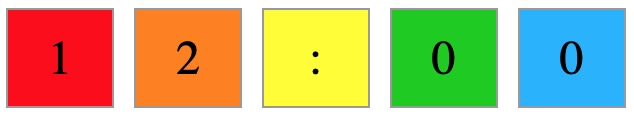Computer animation: military demo
TBD: manual demo
TBD: automatic clock

## Standard time - 5 cubesComputer animation: standard 5 demo

## Standard time - 6 cubesComputer animation: standard 6 demo
TBD: manual demo
TBD: automatic clock

# How does it work?

The question is: Is it possible to make a clock from 4 cubes?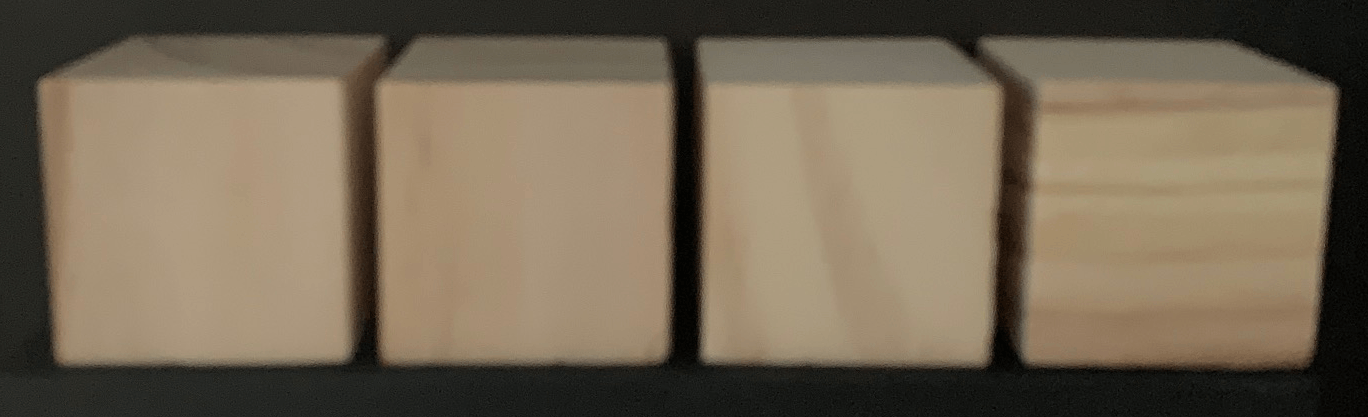A cube has 6 sides. With 4 cubes there are in total 24 sides.

## Standard time - 4 cubes

The standard time ranges from 1:00 to 12:59.

There are 4 positions:
• The first position is blank or '1'.
• The second position can be each digit.
• The third position is a digit from '0' up to and including '5'.
• The fourth position can be each digit.
A possible time is 11:11. This means that there are a least 4 sides with '1' necessary.

The tabel gives the number of occurrences of each digit.

timedigit#
10:0003
11:1114
2:2223
3:3333
4:4443
5:5553
6:x662
7:x772
8:x882
9:x992
1:23blank1
total-28

In total there are 28 digits needed to make all different possible.times. This is four more than the 24 cube sides. It is necessary to reduce the number of digits. Which way can this be done?
• The digits 6 and 9 are special. When 6 is turned 180 degrees you get 9. This means that the 6 can be used as 9. This reduces the number of necessary numbers with two.

• The digits 2 and 5 are also special, but it impossible to turn a cube to make a five from a two or a two from a five. The digits are a line mirroring. This cannot be used to reduce the total.

• The digit 0 is used only once three times. This is in "10:00". Are there ways to display this time in such a way that maximaal two digit 0 are used and that it is still clear that it is 10 o'clock?
Three possible solutions "10: 0", "10:-0" and "10:--".
Each solution reduces the number of used digits with 1 to 25.

• The blank side of the cube is not necessary as it is also possible to just remove the cube from the display. This reduces the number of used digits with 1 to 24.
So based on the number digits it may be possible to make the standard time with 4 cubes.

But is it possible to divide the digit over the cubes in such a way that all possible times can be displayed? Yes, there are several solutions.

The used solution is:Some examples: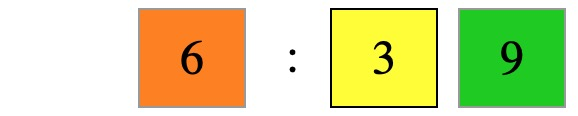Examples with real cubes: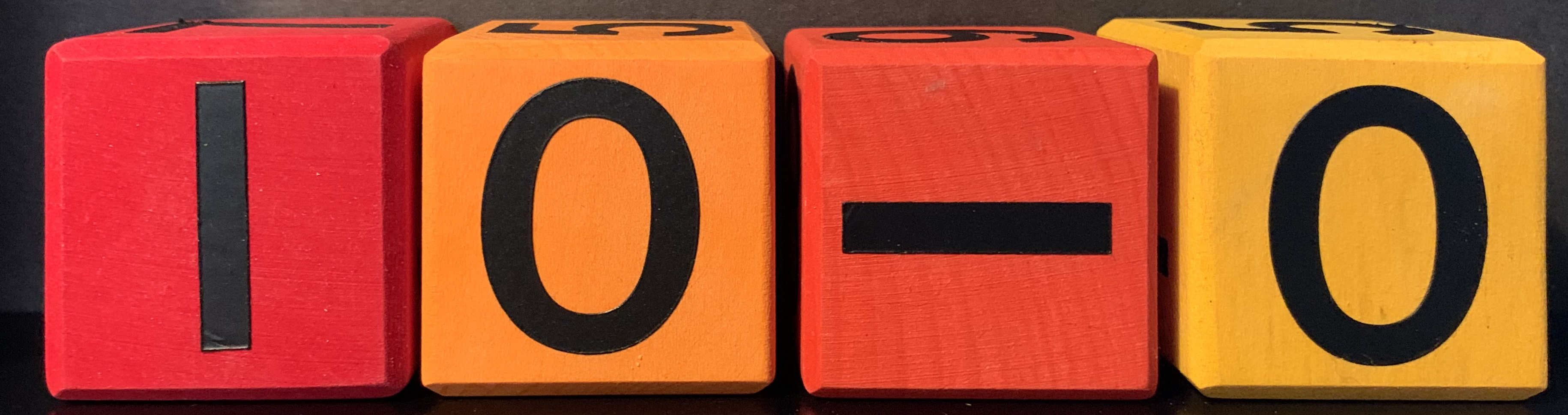## Military time

The military time ranges from 00:00 to 23:59.

There are 4 positions:
• The first position is '0', '1' or '2'.
• The second position can be each digit.
• The third position is a digit from '0' up to and including '5'.
• The fourth position can be each digit.
A possible time is 22:22. This means that there are a least 4 sides with '2' necessary.

The tabel gives the number of occurrences of each digit.

timedigit#
00:0004
11:1114
22:2224
x3:3333
x4:4443
x5:5553
x6:x662
x7:x772
x8:x882
x9:x992
total-29

In total there are 29 digits needed to make all different possible.times. This is five more than the 24 cube sides. It is necessary to reduce the number of digits. Can this be done?
• The digits 6 and 9 are special. When 6 is turned 180 degrees you get 9. This means that the 6 can be used as 9. This reduces the number of necessary numbers with two.

• The digits 2 and 5 are also special, but it impossible to turn a cube to make a five from a two or a two from a five. The digits are a line mirroring. This cannot be used to reduce the total.

• The digit 0 is special as the value is zero. The normal way of presenting 10 o'clock is "10:00", but also "10:--" will be understood. This reduces the number of necessary numbers with another two.
This reduces the number of used digits to 25. So based on the number digits it is not possible to make the military time with 4 cubes.

The chosen solution is to present the time with 5 cubes, whereby the third cube is used to present the semi column.Some examples:Examples with real cubes:## Standard time - 5 cubes

The standard time with 4 cubes does not contain an AM/PM indicator. The most simple way to add AM/PM is to use the standard 4 cubes with one extra cube to indicate AM/PM. With this there are only two sides used on the last cube. The empty sides can be used for "noon" and "midnight", whereby the last may be split into "mid" and "night". The last open position is used for the company name "ap27".Some examples:## Standard time - 6 cubes

The standard time with 6 cubes uses two cubes for AM/PM, one for A or P and one for M. This can be done in combination with the standard time with 4 cubes, but this solution uses the removal of a cube to present the space.
It is preferred to always use all cubes. The space needs be presented with a blank side in that case. The standard time ranges from 12:00 AM to 11:59 PM.

There are 6 positions:
• The first position is blank or '1'.
• The second position can be each digit.
• The third position is a digit from '0' up to and including '5'.
• The fourth position can be each digit.
• The fifth position is a 'A' or 'P'.
• The sixth position is a 'M'.
It was not possible to find a direct solution with 6 cubes with to cover all possible times. So it was necessary to do something special.
• As with the other solutions, the digits 6 and 9 are covered with the only one digit: 6.

• The characters 3 and M are also special. When you turn the 3 90 degrees, you will get the M. So you can cover the 3 and M with only one digit: 3.
Both methods together make it possible to present all possible times with 6 cubes. It is even possible to add something special for midnight and noon. The final solution is to present the time with 6 cubes.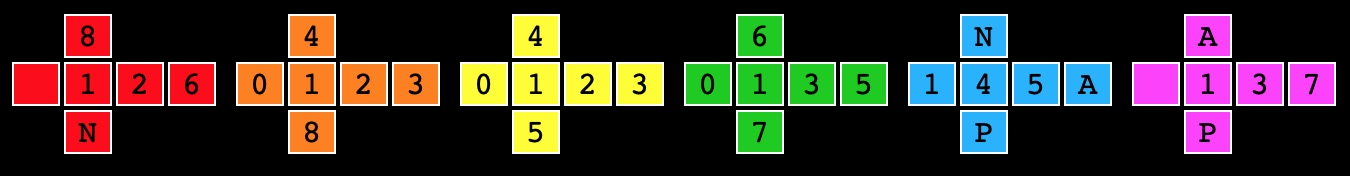Some examples: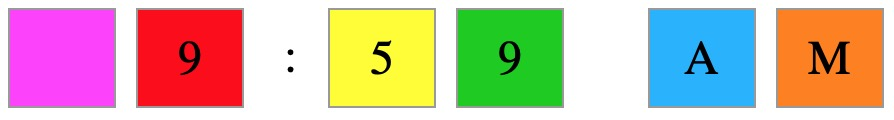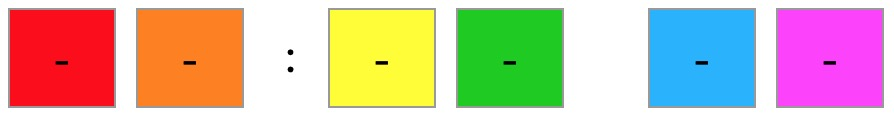Examples with real cubes:# Comparison

standard 4standard 5militarystandard 6
12001200 MID/NIGHT00:001200MN
12011201 AM00:011201AM
12591259 AM00:591259AM
100 100 AM01:00 100AM
101 101 AM01:01 101AM
959 959 AM09:59 959AM
10 010 0 AM10:001000AM
10-010-0 AM10:001000AM
10--10-- AM10:001000AM
10011001 AM10:011001AM
11591159 AM11:591159AM
12001200 NOON12:00-N00N-
12011201 PM12:011201PM
100 100 PM13:00 100PM
11591159 PM23:591159PM